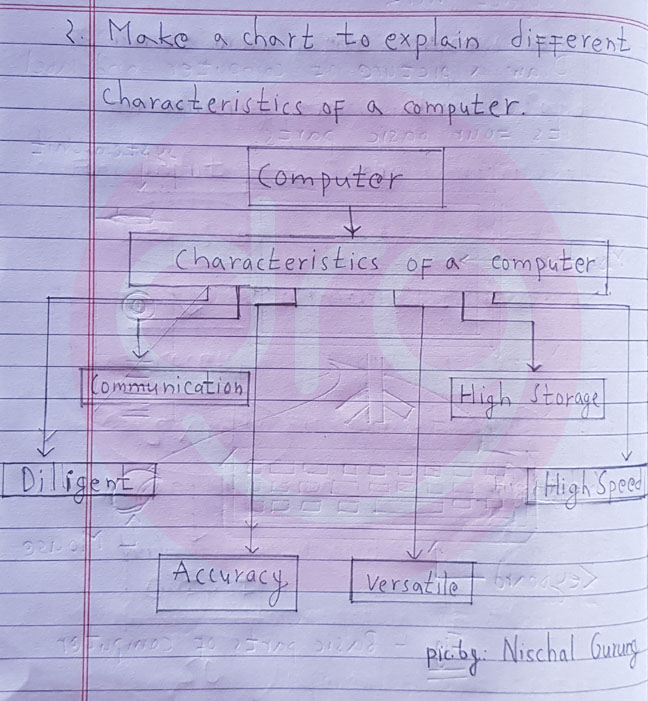Grade IV (4) Notes of Chapter One (1) - An Introduction To Computer.

### EXERCISE:

1. Fill in the blanks with the given words from the box below.
Process, raw data, computare, processing speed, CPU
a. A computer is an electronic machine that process raw data and gives result.
b. The name computer is derived from the Latin word computare.
c. Input, process and output is the basic working principle of a computer.
d. The processing is done by CPU.
e. The time taken by a computer to process the data is called processing speed.

2. Write the characteristics of a computer in a single word.
a. A computer never gets tired or bored while working for a long time. - Diligent
b. A computer does not make any mistake. - Accuracy
c. A computer can perform billions of calculations in a second. - High Speed
d. A computer can perform the several tasks such as drawing pictures, playing games, etc. - Versatile

a. Write any four electronic machines that we use in our daily life.
Answer: The four electronic machines that we use in our daily life are:-
1. Mobile phone.
2. Calculator.
3. Camera.
4. Television.
b. What is a computer?
Answer: A computer is an electronic machine that computes data and produces meaningful information.

c. Which was the first calculating device in the world?
Answer: ABACUS was the first calculating device in the world.

d. What are the four basic parts of the computer?
Answer: The four basic parts of the computer are:-
1. Keyboard
2. Mouse
3. Monitor
4. System Unit.
e. What is the basic working principle of a computer?
Answer: Input, process and output is the basic working principle of a computer.

f. Write down any four characteristics of a computer.
Answer: The four characteristics of a computer are:-
1. Diligent
2. Accuracy
3. High speed
4. Versatile.
g. List any four places where computer are used.
Answer: The four places where computer are used are:-
1. School
2. Office
3. Bank
4. Hospital.
ddddddd
4. Write full form of the following:
a. CPU, b. ATM, c. MHz, d. GHz
a. CPU - Central Processing Unit
b. ATM - Automated + Teller Machine
c. MHz - Mega Hertz
d. GHz - Giga Hertz.

5. Find the computer related words from the following:Computer related words
MOUSE
KEYBOARD
MONITOR
OUTPUT
PROCESSING

### PROJECT WORK:

1. Draw a picture of a computer and label its four basic parts.Basic Pars Of Computer

2. Make a chart to explain different characteristics of a computer.Characteristics of a Computer
3. Draw a picture to show input, process and output for making a glass of Juice.Making of Juice - Input→Process→Output

4. Write down input, process and output to multiply two numbers i.e. 5 and 10.Input→Process→Output

Search Terms/button
Introduction To Computers., Computers Notes of Grade IV-4., Computer Notes Chapter 1/Fill in the blanks., Class 4 Computer Notes., Computers Notes for Class 4., Check All Computer Notes Grade IV-4., Computer Exercise Solution., Question answers of Computer Chapter 1 - introduction to computers alert-info